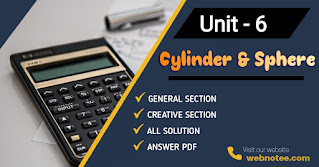### Unit: 6  Mensuration: Cylinder and Sphere

Before starting the discussion about Cylinder and Sphere we have to review the following concept.

### Cylinder

A cylinder is a solid object with three dimensions which holds two parallel bases which is joined by a curved surface at a fixed distance.

Curved Surface Area (CSA) of the cylinder
= area of the rectangle
=  2πrh
= c × h, where c is circumference of base of the cylinder
∴ C.S.A. of the cylinder = 2πrh or c × h

Total surface area (TSA) of the cylinder
= CSA + 2 (area of circular base)
= 2πrh + 2πr²
= 2πr(r+h)
= c(r+h)
Where c is the circumference of base

Volume of Cylinder
= Base area × Height
= A × h
Or
πr²h

### Sphere

A sphere is a solid object, each point of its surface is equidistant from a fixed point inside it. The fixed point is called the centre and the constant distance is called the radius of the sphere. Objects such as globe, football, tennis ball, etc. are examples of spheres.
For a sphere, if radius = R and diameter = d

Surface Area
= 4πr² or πd²

Volume
= 4/3 × πr²

#### For Hemisphere

Curved surface area (C.S.A)
= 2πr²

Total surface area (T.S.A)
= C.S.A + area of circular face
= 2πr² + πr²
= 3πr²

## Mensuration: Cylinder and Sphere Class 10 Maths Solution PDF

NoteScroll the PDF to view all Solution

You are not allowed to post this PDF in any website or social platform without permission.

## Is Class 10 Mathematics Guide Helpful For Student ?

I have published this Notes for helping students who can't solve difficult maths problems. Student should not fully depend on this note for doing all exercises. If you totally depend on this note and simply copy a to z then it may affect your study.

Student should also try to solve some problems themselves. You can use this note as a reference. You should check all the answers carefully because all the answers may not be correct. There may be some minor mistakes in the note, please consider those mistakes.

## How to secure good marks in Mathematics ?

As, you may know I'm also a student. Being a student is not so easy. You have to study and work hard in 8 different subjects. From my point of view most of the student are weak in mathematics. They face difficulties while solving maths problems. I was also facing the same problem when I was in grade 10.

If you also want to secure good marks in mathematics then you should practise them everyday. You should always start your practise from a simple problems. When you solve some simple problems then it will motivates you to solve some other harder problem. Slowly and gradually increase the difficulty of questions day by day.

Maths is not only about practising. You also needs to be clear about the concept of solving the problems. When you get the concept then you can easily solve maths problems which are in similar formats.

You should make the habit of making side notes. It means you should make notes of formulas, tips of solving that problems and the main concept.

When your teacher tries to make the concept clear by giving some random example then all student tries to remember the same example but you shouldn't do that. You should try to relate that concept with your daily life and create your own formula of remembering the concepts.

If you give proper time for your practise with proper method of practising then you'll definitely score a good marks in your Exam. You can also make a time table for your study and read accordingly.

Disclaimer: This website is made for educational purposes. If you find any content that belongs to you then contact us through the contact form. We will remove that content from our as soon as possible.

If you have any queries or wants to give any suggestion then feel free to comment down.

No Comment